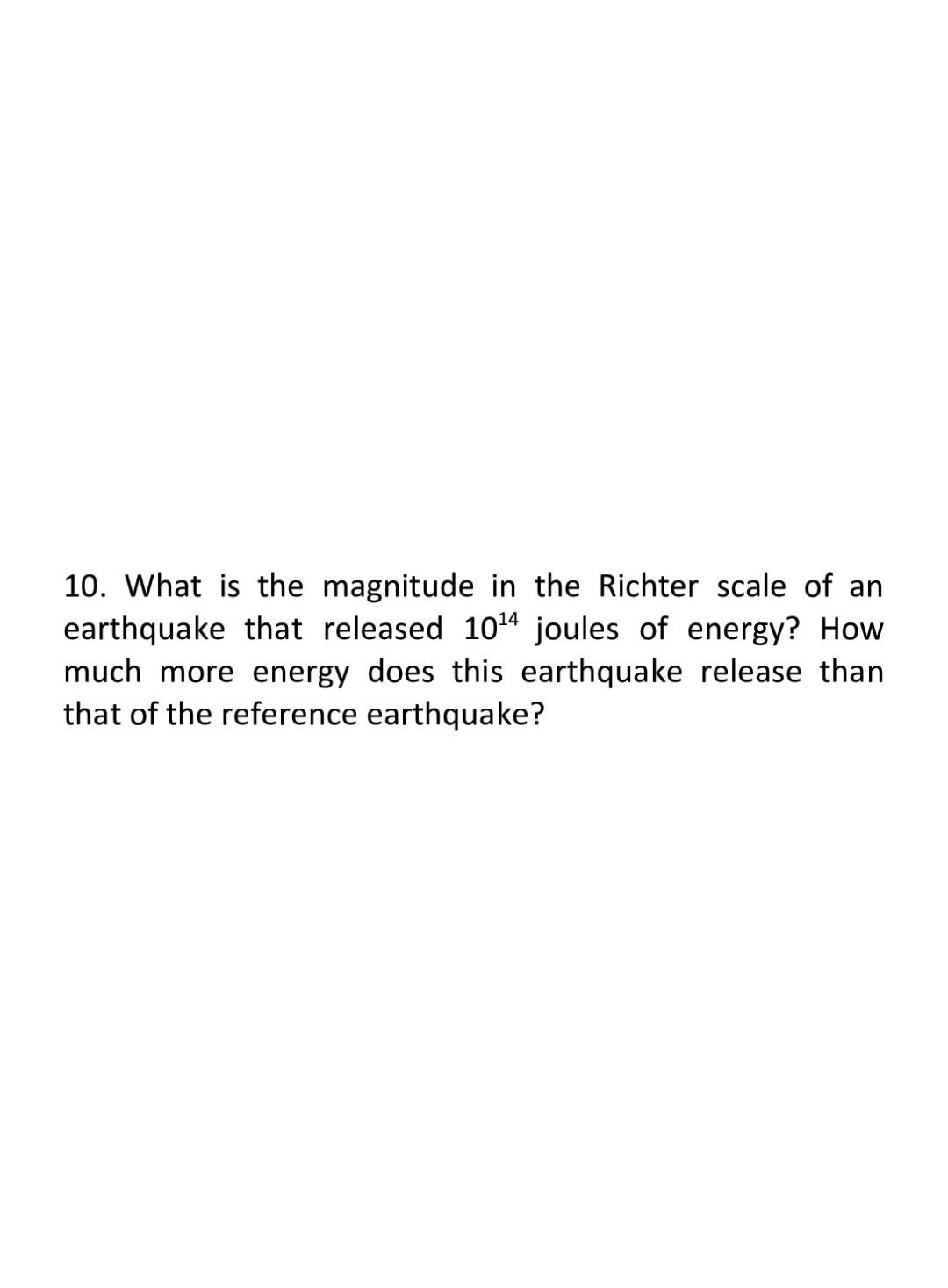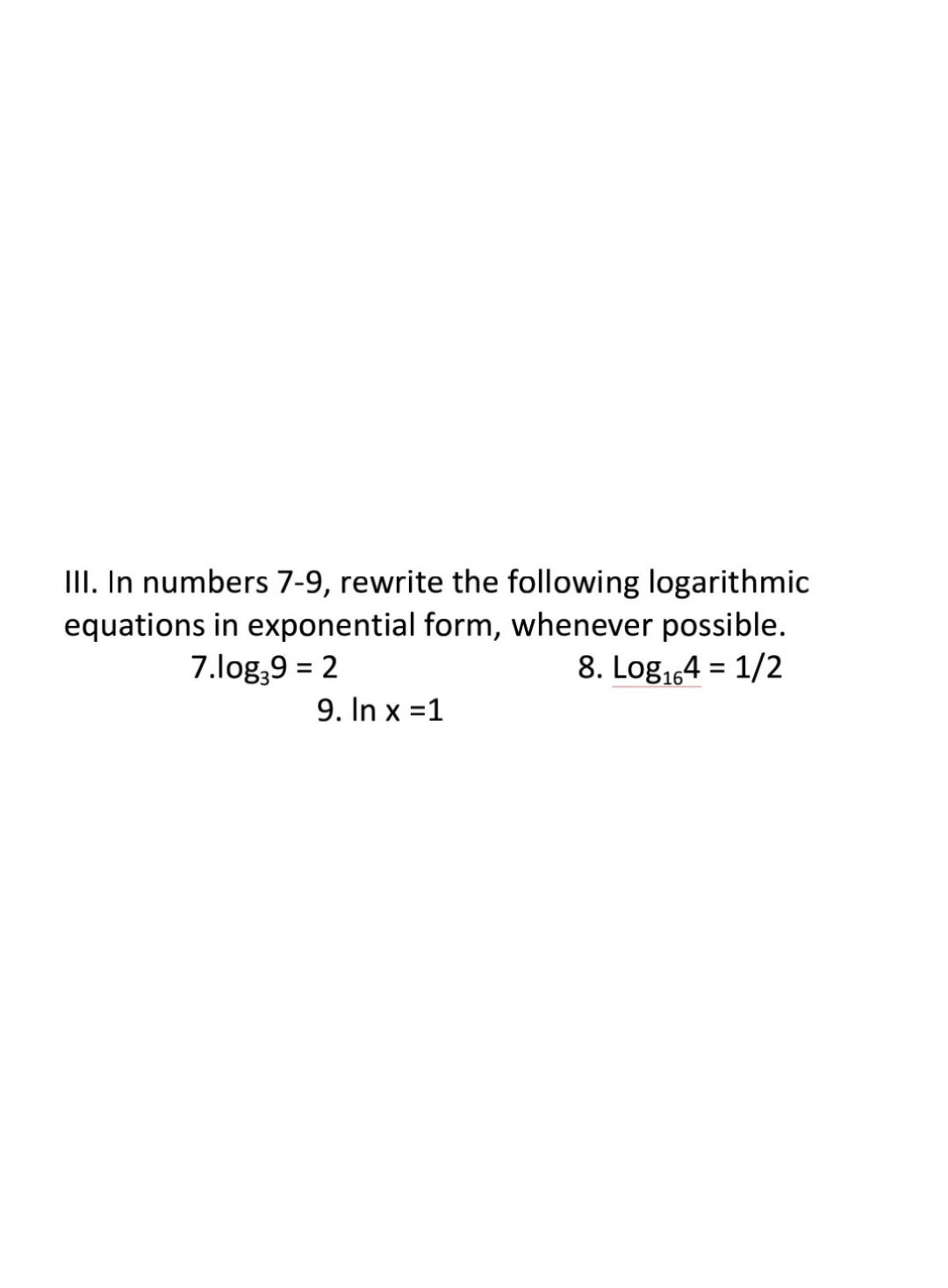Symbol
Problem$1.$ In numbers $1-3,$ find the value of the following logarithmic expressions. $1.$ $log _{3}81$ $2$ $log _{169}13$ $3$ $log _{5}\left(1/5\right)$
Other
SolutionQanda teacher - Mike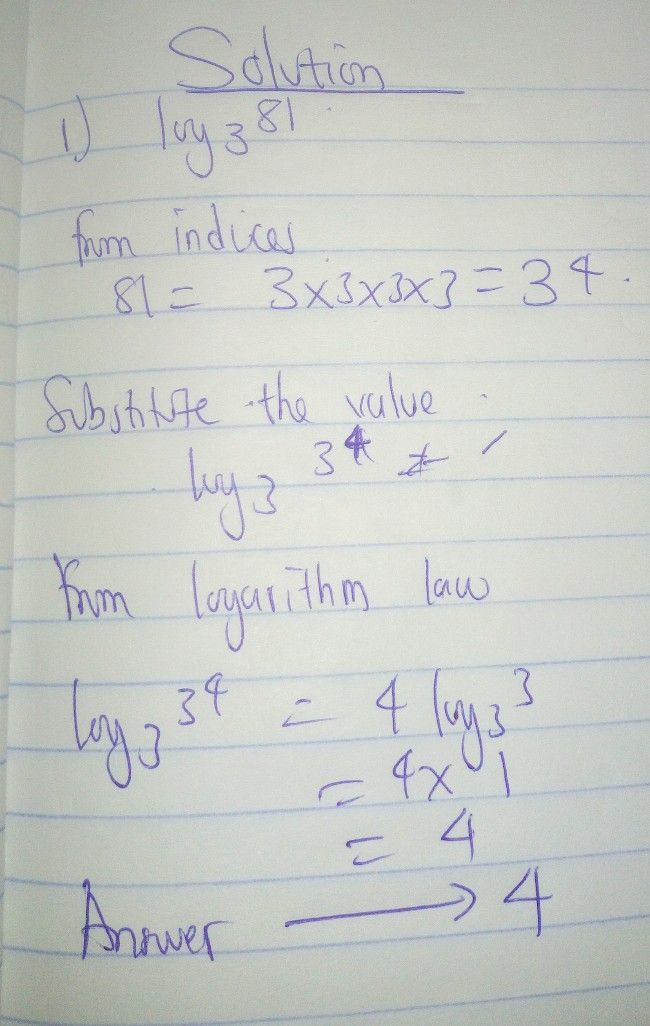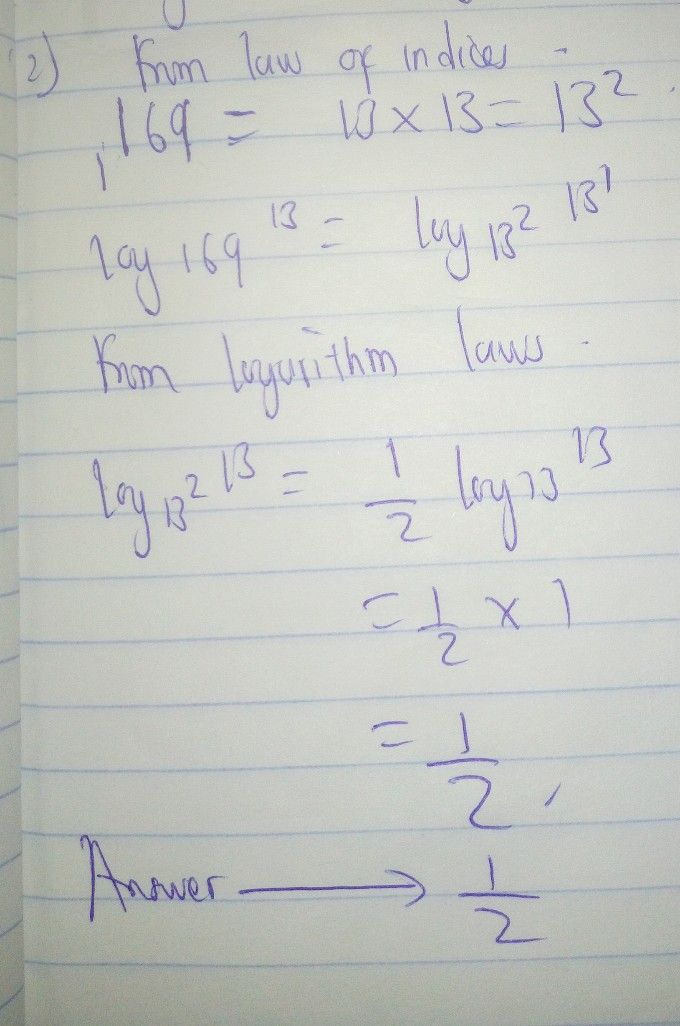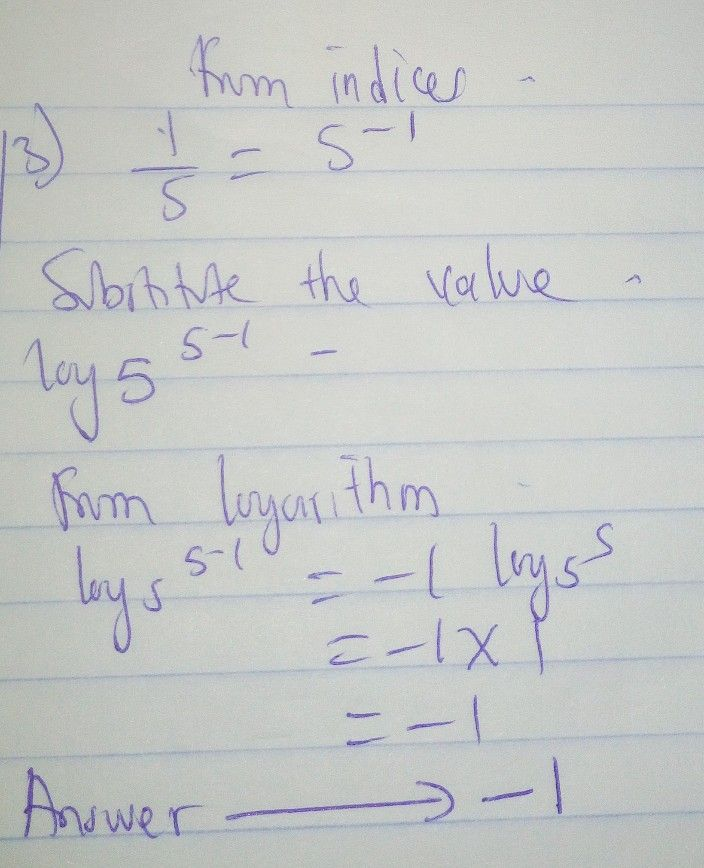you can rate it ok
click on the evaluate button at the top right corner
select solution complete
select 5 StarsStudent